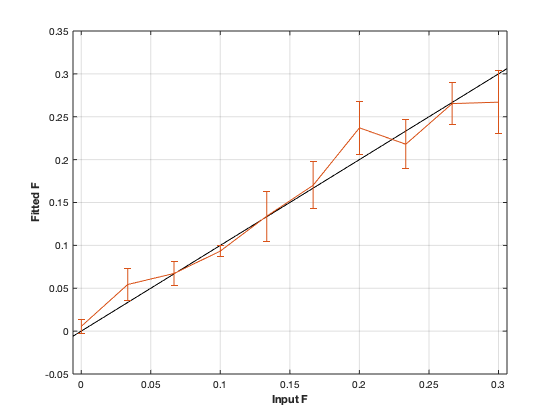# qmt_bssfp : qMT using Balanced Steady State Free Precession acquisition¶## Contents

```% This m-file has been automatically generated using qMRgenBatch(qmt_bssfp)
% Command Line Interface (CLI) is well-suited for automatization
% purposes and Octave.
%
% Please execute this m-file section by section to get familiar with batch
% processing for qmt_bssfp on CLI.
%
%
% Written by: Agah Karakuzu, 2017
% =========================================================================
```

## I- DESCRIPTION

```qMRinfo('qmt_bssfp'); % Describe the model
```
```  qmt_bssfp : qMT using Balanced Steady State Free Precession acquisition
-----------------------------------------------------------------------------------------------------
Assumptions:

Inputs:
MTdata        4D Magnetization Transfer data
(R1map)       1/T1map (optional)

Outputs:
F         Ratio of number of restricted pool to free pool, defined
as F = M0r/M0f = kf/kr.
kr        Exchange rate from the free to the restricted pool
(note that kf and kr are related to one another via the
definition of F. Changing the value of kf will change kr
accordingly, and vice versa).
R1f       Longitudinal relaxation rate of the free pool
(R1f = 1/T1f).
R1r       Longitudinal relaxation rate of the restricted pool
(R1r = 1/T1r).
T2f       Tranverse relaxation time of the free pool (T2f = 1/R2f).
M0f       Equilibrium value of the free pool longitudinal
magnetization.

M0r       Equilibrium value of the restricted pool longitudinal
magnetization.
kf        Exchange rate from the restricted to the free pool.
resnorm   Fitting residual.

Protocol:
MTdata      Array [nbVols x 2]:
Alpha   Flip angle of the RF pulses (degrees)
Trf     Duration of the RF pulses (s)

Options:
RF Pulse
Shape           Shape of the RF pulses.
Available shapes are:
- hard
- gaussian
- gausshann (gaussian pulse with Hanning window)
- sinc
- sinchann (sinc pulse with Hanning window)
- singauss (sinc pulse with gaussian window)
- fermi
Nb of RF pulses Number of RF pulses applied before readout.

Protocol Timing
Fix TR          Select this option and enter a value in the text
box below to set a fixed repetition time.
Fix TR - Trf    Select this option and enter a value in the text
box below to set a fixed free precession time
(TR - Trf).
Prepulse      Perform an Alpha/2 - TR/2 prepulse before each
series of RF pulses.

R1
Use R1map to      By checking this box, you tell the fitting
constrain R1f   algorithm to check for an observed R1map and use
its value to constrain R1f. Checking this box
will automatically set the R1f fix box to true in
the Fit parameters table.
Fix R1r = R1f     By checking this box, you tell the fitting
algorithm to fix R1r equal to R1f. Checking this
box will automatically set the R1r fix box to
true in the Fit parameters table.

Global
G(0)              The assumed value of the absorption lineshape of
the restricted pool.

References:
Please cite the following if you use this module:

In addition to citing the package:
Karakuzu A., Boudreau M., Duval T.,Boshkovski T., Leppert I.R., Cabana J.F.,
Gagnon I., Beliveau P., Pike G.B., Cohen-Adad J., Stikov N. (2020), qMRLab:
Quantitative MRI analysis, under one umbrella doi: 10.21105/joss.02343

Reference page in Doc Center
doc qmt_bssfp

```

## a- create object

```Model = qmt_bssfp;
```

## b- modify options

```         |- This section will pop-up the options GUI. Close window to continue.
|- Octave is not GUI compatible. Modify Model.options directly.```
```Model = Custom_OptionsGUI(Model); % You need to close GUI to move on.
```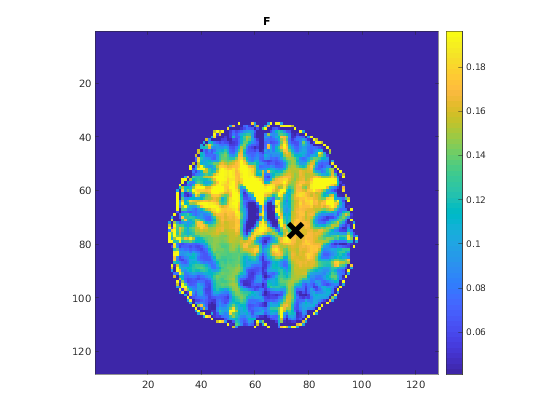## III- FIT EXPERIMENTAL DATASET

```         |- qmt_bssfp object needs 3 data input(s) to be assigned:
|-   MTdata
|-   R1map
```data = struct();
% MTdata.nii.gz contains [128  128    1   16] data.
% R1map.nii.gz contains [128  128] data.
% Mask.nii.gz contains [128  128] data.
```

## b- fit dataset

`           |- This section will fit data.`
```FitResults = FitData(data,Model,0);
```
```=============== qMRLab::Fit ======================
Operation has been started: qmt_bssfp
Elapsed time is 0.168143 seconds.
Operation has been completed: qmt_bssfp
==================================================
```

## c- show fitting results

```         |- Output map will be displayed.
|- If available, a graph will be displayed to show fitting in a voxel.
|- To make documentation generation and our CI tests faster for this model,
we used a subportion of the data (40X40X40) in our testing environment.
|- Therefore, this example will use FitResults that comes with OSF data for display purposes.
|- Users will get the whole dataset (384X336X224) and the script that uses it for demo
via qMRgenBatch(qsm_sb) command.```
```FitResults_old = load('FitResults/FitResults.mat');
qMRshowOutput(FitResults_old,data,Model);
```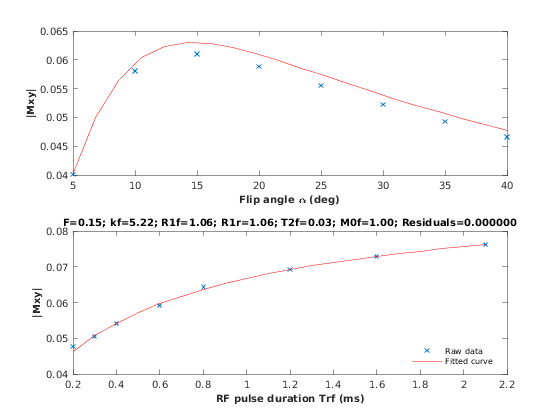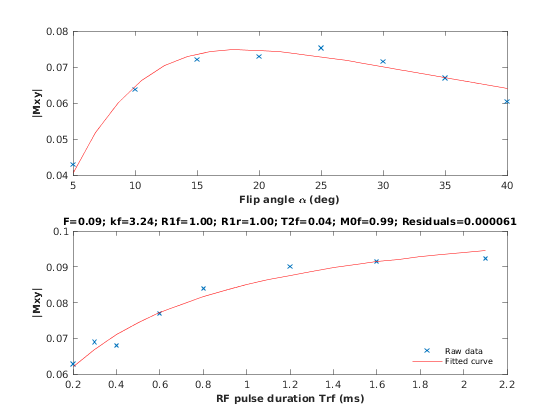## d- Save results

```         |-  qMR maps are saved in NIFTI and in a structure FitResults.mat
that can be loaded in qMRLab graphical user interface
|-  Model object stores all the options and protocol.
It can be easily shared with collaborators to fit their
own data or can be used for simulation.```
```FitResultsSave_nii(FitResults, 'qmt_bssfp_data/MTdata.nii.gz');
Model.saveObj('qmt_bssfp_Demo.qmrlab.mat');
```
```Warning: Directory already exists.
```

## V- SIMULATIONS

`   |- This section can be executed to run simulations for qmt_bssfp.`

## a- Single Voxel Curve

```         |- Simulates Single Voxel curves:
(1) use equation to generate synthetic MRI data
(3) fit and plot curve```
```      x = struct;
x.F = 0.1;
x.kr = 30;
x.R1f = 1;
x.R1r = 1;
x.T2f = 0.04;
x.M0f = 1;
% Set simulation options
Opt.SNR = 50;
Opt.Method = 'Analytical equation';
Opt.ResetMz = false;
% run simulation
figure('Name','Single Voxel Curve Simulation');
FitResult = Model.Sim_Single_Voxel_Curve(x,Opt);
```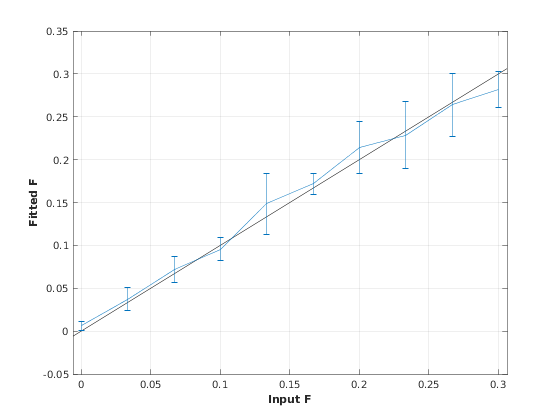## b- Sensitivity Analysis

```         |-    Simulates sensitivity to fitted parameters:
(1) vary fitting parameters from lower (lb) to upper (ub) bound.
(2) run Sim_Single_Voxel_Curve Nofruns times
(3) Compute mean and std across runs```
```      %              F             kr            R1f           R1r           T2f           M0f
OptTable.st = [0.1           30            1             1             0.04          1]; % nominal values
OptTable.fx = [0             1             1             1             1             1]; %vary F...
OptTable.lb = [0.0001        0.0001        0.2           0.2           0.01          0.0001]; %...from 0.0001
OptTable.ub = [0.3           1e+02         3             3             0.2           2]; %...to 0.3
% Set simulation options
Opt.SNR = 50;
Opt.Method = 'Analytical equation';
Opt.ResetMz = false;
Opt.Nofrun = 5;
% run simulation
SimResults = Model.Sim_Sensitivity_Analysis(OptTable,Opt);
figure('Name','Sensitivity Analysis');
SimVaryPlot(SimResults, 'F' ,'F' );
```